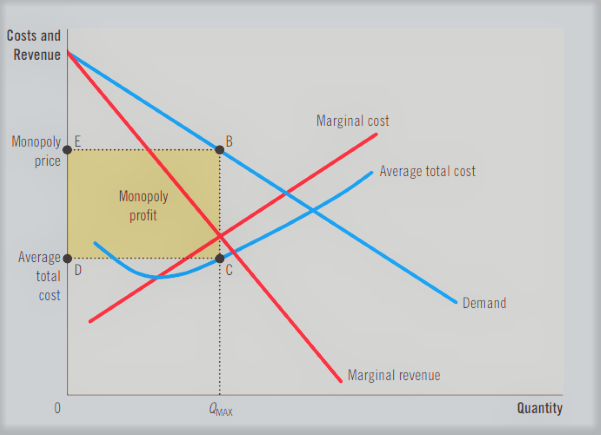# MCQs Microeconomics of Monopoly

## What is Monopoly

A scenario known as monopoly occurs when there is only one seller in the market. The monopoly case is the polar opposite of perfect competition in conventional economic analysis. The industry's downward-sloping demand curve is, by definition, the demand curve that the monopolist faces. As a result, the monopolist has considerable control over the price it sets, i.e., it sets prices rather than being a price taker.

### Why There Are Monopolies

If a company sells its product exclusively and there are no close substitutes, it has a monopoly. Barriers to entry are the monopoly's primary cause: Because other businesses are unable to enter the market and compete with it, a monopoly continues to be the only seller there. Entry barriers generally come from three different places:

• Monopoly resources: One company controls a crucial resource needed for production.
• Government regulation: The government grants one company the sole authority to create a good or service.
• The manufacturing process: Compared to a larger number of enterprises, a single firm can create output at a cheaper cost.

### The Monopolist’s ProfitThe profit of the monopolistic corporation is equal to the area of the box BCDE. Price less average total cost, which represents profit per unit sold, is the height of the box (BC). The quantity sold is indicated by the box width (DC).

### Mathematical Approach to solve Profit maximization in Monopoly

Calculus is used to get the mathematical answer for maximizing profits. Finding a function's greatest level involves finding the first derivative and setting it to zero. Remember that the monopolist faces an inverse demand function with the formula P = 100 - Qd and unit costs of Rs 50 per ounce.

max π = TR – TC

= P(Q)Q – C(Q)

= (150 – Q) Q – 50Q

= 150Q – Q2 – 50Q

∂π/∂Q= 150 – 2Q – 50 = 0

2Q = 100

Q* = 50 million ounces of chemical

The profit-maximizing price is found by substituting Q* into the inverse demand equation:

P* = 150 – Q* = 150 – 50 = 100 PKR/ounce of chemical.

The maximum profit level can be found by substitution of P* and Q* into the profit equation:

π = TR – TC = P(Q)Q – C(Q) = 100*50 – 50*50 = 50*50 = 2500 million PKR.

# The Market Forces of Supply and Demand

#### Monopoly Quiz

##### Please fill the above data!

Generated By Html Quiz Generator

coin :  0